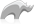##### [ array ]

Type

An array is an object that can hold multiple values which are referenced by an index position. An array can be thought of as a series of numbered variables or as a list. Array elements are indexed from 1 and are always sequential. Arrays can hold values of any other data type. Arrays can hold integers, decimals, strings, pairs, or even other arrays or maps. The size of an array is only limited by the amount of memory that is available.

An array is a random access container. Elements can be quickly accessed by providing the position of the element to retrieve. The first element of an array is at position one. Zero is an invalid position within an array.

Arrays are dynamically sized. Inserting an element into an array will cause the array to grow by one. Removing an element will shrink the array by one.

Arrays provide quick insertion at the end (constant time), but inserting an element at any other position will result in all subsequent elements being shifted down (making the operation O(n)). Because of this, if insertions at the beginning are required, an array might not be the best choice of container (a [list] might perform better).

• Syntax
• Methods
• Traits
``array(element_1, element_2, ..., element_n)``
###### Has methods:
• `array->oncompare(array::array)`
• `array->oncompare(n::void)`
• `array->oncompare(n::null)`
• `array->oncreate()`
• `array->get(p0::integer)`
• `array->get=(p0, p1::integer)`
• `array->size()`
• `array->asstaticarray()`
• `array->insert(p0)`
• `array->insert(p0, p1::integer)`
• `array->merge(p0::array, p1::integer, p2::integer, p3::integer)`
• `array->merge(p0::staticarray, p1::integer, p2::integer, p3::integer)`
• `array->merge(VALUE::trait_forEach)`
• `array->merge(VALUE::trait_forEach, pos::integer)`
• `array->merge(VALUE::trait_forEach, pos::integer, mpos::integer)`
• `array->remove()`
• `array->remove(p0::integer, p1::integer)`
• `array->remove(pos::integer)`
• `array->removeall()`
• `array->removeall(matching)`
• `array->reserve(p0::integer)`
• `array->asString()`
• `array->+(rhs::trait_forEach)`
• `array->contains(VALUE)`
• `array->count(VALUE)`
• `array->exchange(left::integer, right::integer)`
• `array->find(VALUE)`
• `array->findposition(crit, offset::integer = ?)`
• `array->findindex(VALUE)`
• `array->forEach()`
• `array->forEachPair()`
• `array->forEachKey()`
• `array->keys()::staticarray`
• `array->reverse()`
• `array->sort(-descending::boolean = ?, -ascending::boolean = ?)`
• `array->sort(ascending::boolean)`
• `array->values()::staticarray`
• `array->serializationElements()`
• `array->acceptDeserializedElement(d::serialization_element)`
• `array->speak()`
###### Has traits:
• `any`
• `trait_array`
• `trait_asString`
• `trait_backContractible`
• `trait_backEnded`
• `trait_backExpandable`
• `trait_contractible`
• `trait_doubleEnded`
• `trait_endedFullyMutable`
• `trait_expandable`
• `trait_finiteForEach`
• `trait_finite`
• `trait_forEach`
• `trait_frontContractible`
• `trait_frontEnded`
• `trait_frontExpandable`
• `trait_fullyMutable`
• `trait_keyedFinite`
• `trait_keyedMutable`
• `trait_keyed`
• `trait_positionallyKeyed`
• `trait_queriable`
• `trait_serializable`
• `trait_setOperations`
• Beginner

### To create an array of the days of the week:

Use the [array] type with each of the names of the days of the week as parameters. The following example stores an array with the names of the days of the week in a variable named daysOfTheWeek. We then retrieve an element from the variable using its index.

Code

``````local(daysOfTheWeek) = array('Sunday', 'Monday', 'Tuesday', 'Wednesday', 'Thursday', 'Friday', 'Saturday')
daysOfTheWeek->get(2)``````

Result

`Monday`Please note that periodically LassoSoft will go through the notes and may incorporate information from them into the documentation. Any submission here gives LassoSoft a non-exclusive license and will be made available in various formats to the Lasso community.